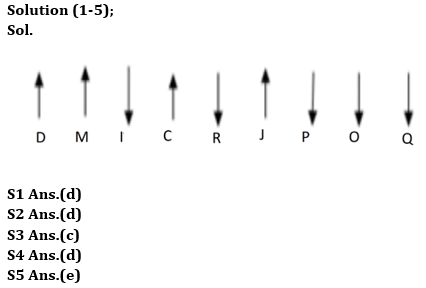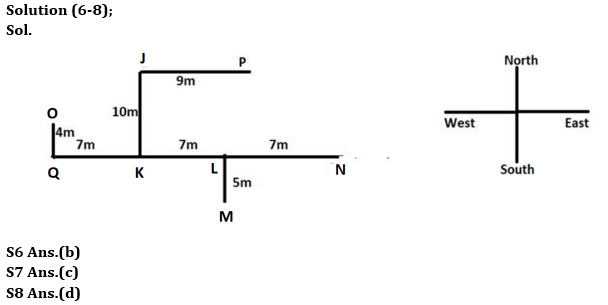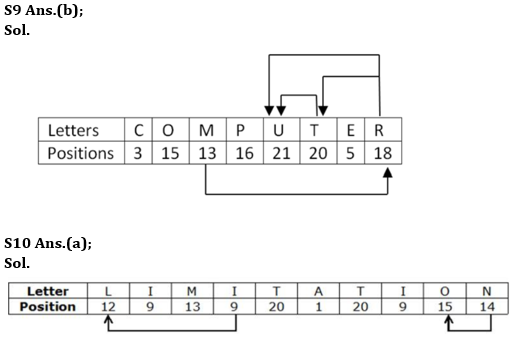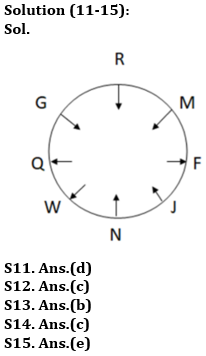Latest Banking jobs   »

# Reasoning Quiz For RBI Grade B Phase 1 2023 -30th April

Directions (1-5): Study the following data carefully and answer the questions accordingly.
J, I, O, D, C, R, P, Q, and M are sitting in a straight line facing the directions either north or south. Only three people are sitting to the right of J. J faces the north direction. I sit three places away from J in the left direction. I and J face opposite directions. I is sitting two places away from R who is not sitting at any of the extreme ends. R is sitting just right of C. C is sitting two places away from M. Both the immediate neighbors of I face the same direction. O is sitting third to the left of R. D is sitting to the right of R. P does not sit at any of the extremes ends of the line. D and C face the same directions. J is sitting to the right of Q. Both P and O face the opposite direction of M.

Q1. If I and J interchange their places, then who will be sitting third to the right of Q?
(a) P
(b) C
(c) M
(d) I
(e) None of these

Q2. How many persons are sitting between the one, who is sitting third from the left end and the one who is sitting just right of O?
(a) One
(b) Two
(c) More than three
(d) Three
(e) None

Q3. What is the position of C with respect to D?
(a) Third to the right of immediate neighbor of D
(b) Fourth to the left
(c) Third to the right
(d) Second to the left
(e) None of these

Q4. Who is sitting in the middle of the line?
(a) The one, who is sitting fourth to the left of M
(b) The one, who is sitting between C and P
(c) The one, who is sitting third to the left of I
(d) The one, who is sitting second to the right of P
(e) None of these

Q5. Find the odd one.
(a) D
(b) C
(c) M
(d) J
(e) O

Direction (6-8): Read the following information carefully and answer the questions which follow.
Point J is 10m to the north of point K which is 7m to the west of point L. Point M is 5m to the south of Point L. Point P is 9m to the east of Point J. Point L is the midpoint between Point K and Point N, point N being towards the East of point K. Point O is 4m to the north of Point Q. Point K lies exactly between Point L and Point Q, point Q being towards the West of point K.

Q6. What is the direction of Point J with respect to Point M?
(a) North-east
(b) North-west
(c) South-east
(d) South-west
(e) None of these

Q7. If point R is 5m south of point K then what is the total distance between Point R and Point N and Point R is in which direction from Point O?
(a) 22m, north-west
(b) 24m, north-east
(c) 19m, south-east
(d) 15m, north
(e) None of these

Q8. Which of the following points lie in the straight line?
(a) J, K and L
(b) O, Q and P
(c) K, L and M
(d) Q, K and N
(e) None of these

Q9. How many such pairs of letters are there in the word COMPUTER each of which has as many letters between them in the word in both forward and backward directions as in the English alphabet?
(a) Two
(b) Four
(c) None
(d) Three
(e) Five

Q10. How many such pairs of letters are there in the word ‘LIMITATION’ each of which has as many letters between them in the word as in the English alphabet (Both forward and backward)?
(a) Two
(b) One
(c) None
(d) Three
(e) More than three

Direction (11-15): Study the following data carefully and answer the questions accordingly.
Eight people are sitting around a circular table. Some of them are facing inside and some are facing outside. R sits fourth to the right of N. F sits second to the left of R, who is facing inside. W sits immediate left of N. At least one person sits between F and W when counted from both sides. G who is not a neighbor of F, sits second to the right of W. Q is not a neighbor of F and faces the opposite direction of N. Immediate neighbors of Q face the opposite direction. G does not sit third to the right of F who faces the opposite direction of J. One person sits between J and M who is not a neighbor of N and faces the opposite direction of Q.

Q11. Who among the following sits third to the right of F?
(a) N
(b) Q
(c) G
(d) W
(e) None of these

Q12. Who is a neighbor of R?
(a) Q
(b) J
(c) G
(d) N
(e) F

Q13. How many people face inside?
(a) Four
(b) Five
(c) Two
(d) Three
(e) None of these

Q14. Find the incorrect statement/statements from the following.
1. Two people sits between G and N when counted right of G.
2. One person sits between F and M, when counted left of M.
3. J and W face the same direction.
(a) Only 1
(b) Both 1 and 3
(c) Both 2 and 3
(d) Only 3
(e) All are correct

Q15. Four of the following five are similar in a certain pattern, who among the following is not following the pattern?
(a) G
(b) M
(c) J
(d) N
(e) Q

Solutions## FAQs

### What is the selection process of the Bank Clerk?

There are 4 sections in the RBI Grade B Phase 1 Exam i.e. English Language, General Awareness, Quantitative Aptitude & Reasoning.

#### Congratulations!Union Budget 2023-24: Free PDF